# GED Math : Word Problems in Algebra

## Example Questions

← Previous 1 3 4

### Example Question #1 : Word Problems In Algebra

Which of the following phrases can be written as the algebraic expression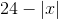?

The absolute value of a number decreased by twenty-four

The opposite of the difference of a number and twenty-four

Twenty-four decreased by the absolute value of a number

Twenty-four decreased by the opposite of a number

Twenty-four decreased by the absolute value of a number

Explanation:is twenty-four decreased by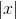, which is the absolute value of a number, sois "twenty-four decreased by the absolute value of a number."

### Example Question #1 : Word Problems In Algebra

Which of the following phrases can be written as the algebraic expression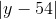?

The absolute value of the difference of a number and fifty-four

The opposite of the difference of a number and fifty-four

The opposite of the product of a number and negative fifty-four

The absolute value of the product of a number and negative fifty-four

The absolute value of the difference of a number and fifty-four

Explanation:is the absolute value of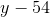, which is the difference of a number and fifty-four; therefore,is "the absolute value of the difference of a number and fifty-four."

### Example Question #3 : Word Problems In Algebra

Which of the following phrases can be written as the algebraic expression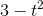?

The square of a number decreased by three

Three decreased by the square of a number

The square of the difference of a number and three

The square of the difference of three and a number

Three decreased by the square of a number

Explanation:is three decreased by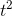, which is the square of a number; therefore,is three decreased by the square of a number.

### Example Question #2 : Word Problems In Algebra

Which of the following phrases can be written as the algebraic expression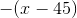?

The absolute value of the difference of forty-five and a number

The absolute value of the difference of a number and forty-five

The opposite of the difference of a number and forty-five

The opposite of the difference of forty-five and a number

The opposite of the difference of a number and forty-five

Explanation:is the opposite of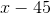, which is the difference of a number and forty-five; therefore,is the opposite of the difference of a number and forty-five.

### Example Question #1 : Word Problems In Algebra

Which of the following phrases can be written as the algebraic expression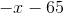?

The absolute value of the difference of a number and sixty-five

Sixty-five subtracted from the opposite of a number

The opposite of the difference of a number and sixty-five

Sixty-five subtracted from the absolute value of a number

Sixty-five subtracted from the opposite of a number

Explanation:is sixty-five subtracted from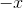, which is the opposite of a number; therefore,is "sixty-five subtracted from the opposite of a number."

### Example Question #2 : Word Problems In Algebra

Which of the following phrases can be written as the algebraic expression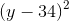?

The square of the difference of a number and thirty-four

Twice the difference of a number and thirty-four

The square of the difference of thirty-four and a number

Twice the difference of thirty-four and a number

The square of the difference of a number and thirty-four

Explanation:is the square of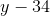, which is the difference of a number and thirty-four. Therefore,is the difference of a number and thirty-four.

### Example Question #4 : Word Problems In Algebra

A broken television cost $1.25 after a 99% discount. What was the original price? Possible Answers:$2.74

$250$125

$12.50$2.49

$125 Explanation: Let x be the original price. If the original price was given a discount, the value of the percent discount must be subtracted from the original price.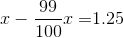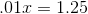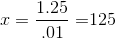### Example Question #1 : Word Problems In Algebra Which of the following phrases can be represented by the algebraic expression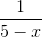? Possible Answers: Five decreased by the reciprocal of a number Five less than by the reciprocal of a number The reciprocal of the difference of five and a number The reciprocal of the difference of a number and five Correct answer: The reciprocal of the difference of five and a number Explanation:is the reciprocal of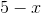, which is the difference of five and a number. Therefore,is "the reciprocal of the difference of five and a number". ### Example Question #696 : Ged Math Sixty-four coins, all dimes and nickels, total$5.15. How many of the coins are dimes?Explanation:

Letbe the number of dimes. Then there are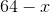nickels.

An equation can be set up and solved forfor the amount of money: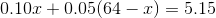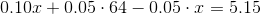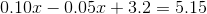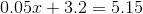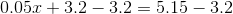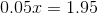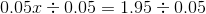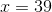, the number of dimes.

### Example Question #697 : Ged Math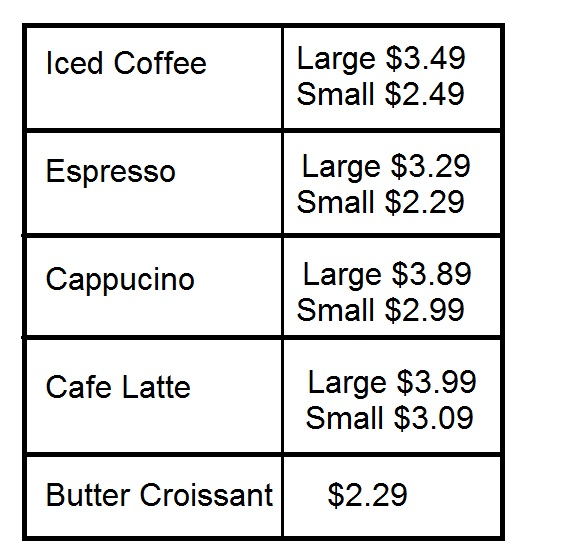Above is the menu for a coffee shop; there is no sales tax charged on purchases.

Greg is throwing a party and wants to buy eight large cappucinos, one for each attendee. He wants to buy all the butter croissants he can without spending more than \$80 total. How many croissants will he be able to buy?Explanation:

Eight large cappucinos will cost Greg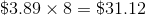.

This leaves him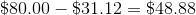to buy croissants, which cost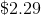.

Letbe the number of croissants he buys. Then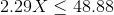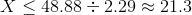Greg can buy up to 21 croissants.

← Previous 1 3 4